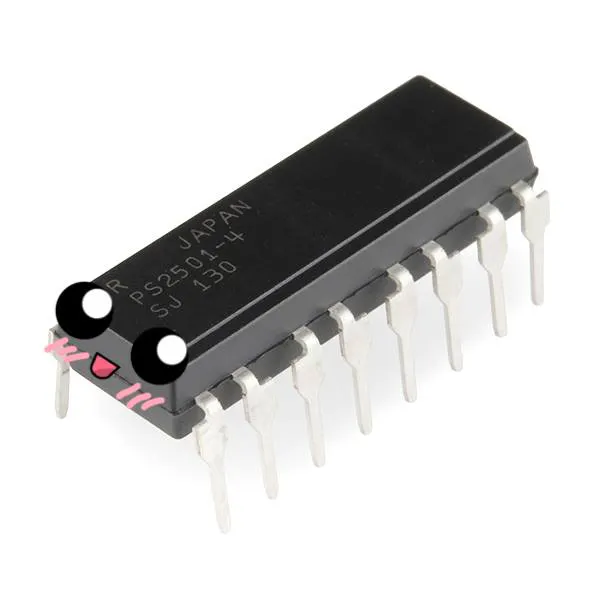# IoT Christmas Tree Topper

IntermediateFull instructions provided4 hours4,973

## Things used in this project

### Hardware components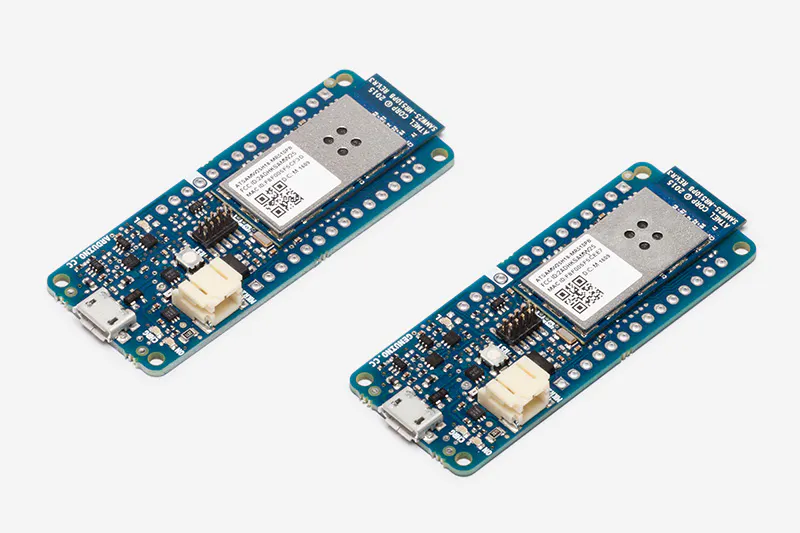Arduino MKR1000
×1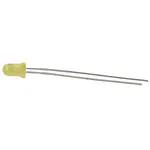3 mm LED: Yellow
×16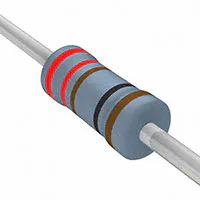Resistor 221 ohm
×4Jumper wires (generic)
×8
 Female header 8 position 2 row (2*4)
×1
 Polystyrene Star
×1

### Software apps and online servicesArduino IDEBlynk

### Hand tools and fabrication machinesSoldering iron (generic)Hot glue gun (generic)

## Schematics

### The circuit of the star display

I know it's not that professional, but it is quite difficult to use a star shape in circuit diagram editors. P1-P4 and N1-N4 lines are not connected to each other. The yellow dots are LEDs, the red wires are positive, the black wires are
negative lines. Solder the LEDs accordingly. Each line is connected to 4 LEDs. The numbers refer to the variables in the code/pins of the MCU. I think it's easy to understand, but if you have difficulties contact me and if it is required I will think of something else.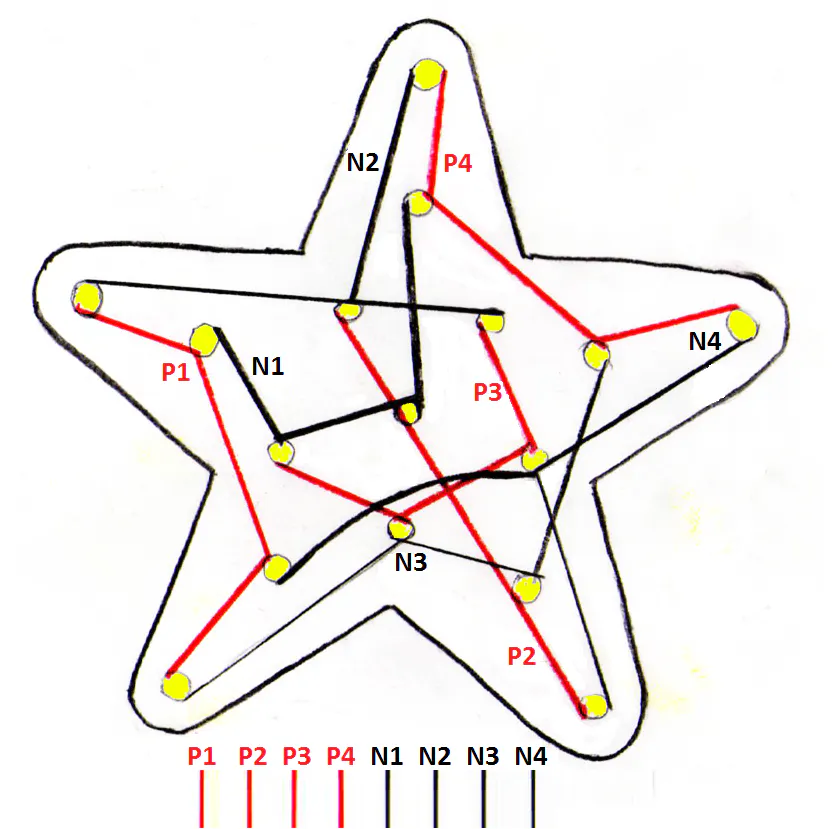### IDs of the LEDs

These IDs are used to refer the LEDs in the plot (array in the code).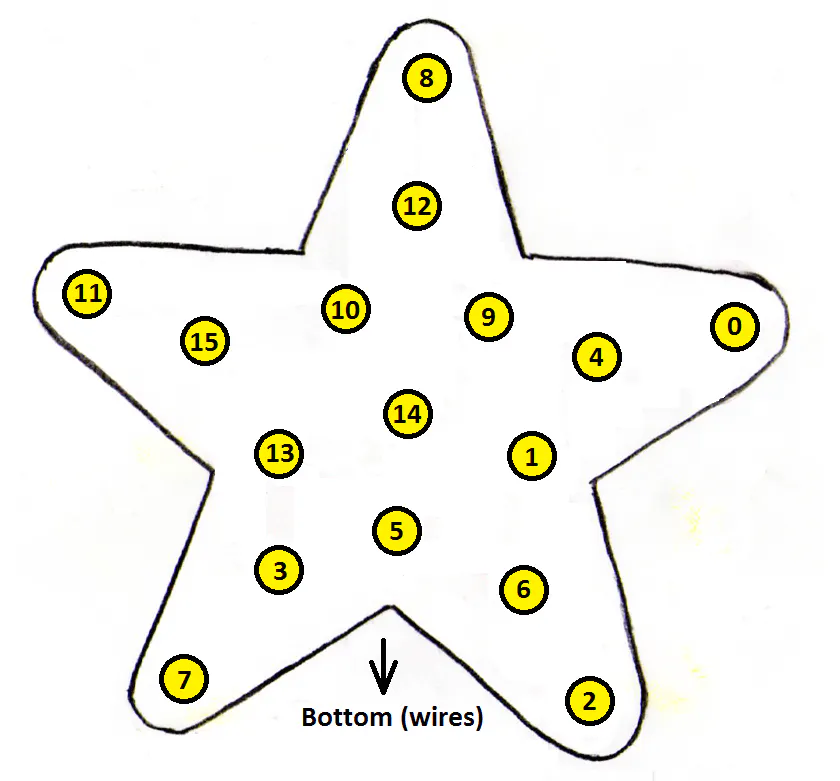## Code

### Star controller code

Arduino
This code is made for Arduino MKR1000. You just upload it to the MCU after you connect the star display. Use the description to connect the display.
```/**************************************************************
* This code controls a star shaped DIY display using an
*
* This project is made for "The Arduino Internet of Holiday Things"
* https://www.hackster.io/contests/arduino-holiday-contest
*
* author: Balázs Simon
*
**************************************************************/

#include <SPI.h>
#include <WiFi101.h>
#include <BlynkSimpleMKR1000.h>

#define P4 2
#define P3 3
#define P2 4
#define P1 5
#define N4 6
#define N3 7
#define N2 8
#define N1 9
#define PLOT_LENGTH 256

// You should get Auth Token in the Blynk App.
// Go to the Project Settings (nut icon).
char auth[] = "YourAuthToken";

// Set password to "" for open networks.
char ssid[] = "YourNetworkName";

int lightingMode = 1;
int changeSpeed = 1;

char plot[PLOT_LENGTH];
int currentStep = 0;

unsigned long lastLEDUpdateTime = 0;

void setup() {

pinMode(P1, OUTPUT);
pinMode(P2, OUTPUT);
pinMode(P3, OUTPUT);
pinMode(P4, OUTPUT);
pinMode(N1, OUTPUT);
pinMode(N2, OUTPUT);
pinMode(N3, OUTPUT);
pinMode(N4, OUTPUT);

for (int i = 0; i < PLOT_LENGTH; i++) {
for (int j = 0; j < 16; j++) {
plot[j][i] = 255;
}
}
}

void loop() {

//Star screen updating based on the "plot"
for (int i = 0; i < 256; i++) {
int j = 0;
digitalWrite(N4, HIGH);
plot[j++][currentStep] > i ? digitalWrite(P1, HIGH) : digitalWrite(P1, LOW);
plot[j++][currentStep] > i ? digitalWrite(P2, HIGH) : digitalWrite(P2, LOW);
plot[j++][currentStep] > i ? digitalWrite(P3, HIGH) : digitalWrite(P3, LOW);
plot[j++][currentStep] > i ? digitalWrite(P4, HIGH) : digitalWrite(P4, LOW);
digitalWrite(N1, LOW);

delayMicroseconds(20);

digitalWrite(N1, HIGH);
plot[j++][currentStep] > i ? digitalWrite(P1, HIGH) : digitalWrite(P1, LOW);
plot[j++][currentStep] > i ? digitalWrite(P2, HIGH) : digitalWrite(P2, LOW);
plot[j++][currentStep] > i ? digitalWrite(P3, HIGH) : digitalWrite(P3, LOW);
plot[j++][currentStep] > i ? digitalWrite(P4, HIGH) : digitalWrite(P4, LOW);
digitalWrite(N2, LOW);

delayMicroseconds(20);

digitalWrite(N2, HIGH);
plot[j++][currentStep] > i ? digitalWrite(P1, HIGH) : digitalWrite(P1, LOW);
plot[j++][currentStep] > i ? digitalWrite(P2, HIGH) : digitalWrite(P2, LOW);
plot[j++][currentStep] > i ? digitalWrite(P3, HIGH) : digitalWrite(P3, LOW);
plot[j++][currentStep] > i ? digitalWrite(P4, HIGH) : digitalWrite(P4, LOW);
digitalWrite(N3, LOW);

delayMicroseconds(20);

digitalWrite(N3, HIGH);
plot[j++][currentStep] > i ? digitalWrite(P1, HIGH) : digitalWrite(P1, LOW);
plot[j++][currentStep] > i ? digitalWrite(P2, HIGH) : digitalWrite(P2, LOW);
plot[j++][currentStep] > i ? digitalWrite(P3, HIGH) : digitalWrite(P3, LOW);
plot[j++][currentStep] > i ? digitalWrite(P4, HIGH) : digitalWrite(P4, LOW);
digitalWrite(N4, LOW);

delayMicroseconds(20);
}

if (millis() - lastLEDUpdateTime > changeSpeed) {

//Moving to the next image in the plot

lastLEDUpdateTime = millis();
currentStep++;

if (currentStep == PLOT_LENGTH) {
currentStep = 0;
}
}
Blynk.run();
}

//Speed slider
BLYNK_WRITE(V1)
{
changeSpeed = param.asInt();
}

//Creating "Simple on" plot
BLYNK_WRITE(V2)
{
if (lightingMode != 1) {
for (int i = 0; i < PLOT_LENGTH; i++) {
for (int j = 0; j < 16; j++) {
plot[j][i] = 255;
}
}

lightingMode = 1;
Blynk.virtualWrite(V1, changeSpeed);
Blynk.virtualWrite(V0, lightingMode);
}
}

//Creating "Flashing" plot
BLYNK_WRITE(V3)
{
if (lightingMode != 2) {
for (int i = 0; i < PLOT_LENGTH / 2; i++) {
for (int j = 0; j < 16; j++) {
plot[j][i] = 255;
}
}

for (int i = PLOT_LENGTH / 2; i < PLOT_LENGTH; i++) {
for (int j = 0; j < 16; j++) {
plot[j][i] = 0;
}
}

lightingMode = 2;
Blynk.virtualWrite(V1, changeSpeed);
Blynk.virtualWrite(V0, lightingMode);
}
}

BLYNK_WRITE(V4)
{
if (lightingMode != 3) {
for (int i = 0; i < PLOT_LENGTH; i++) {
for (int j = 0; j < 16; j++) {
if (i == 0) {
plot[j][i] = 255;
}
else if (i * 2 < PLOT_LENGTH) {
plot[j][i] = PLOT_LENGTH - 2 * i;
}
else {
plot[j][i] = 2 * i - PLOT_LENGTH;
}
}
}
lightingMode = 3;
Blynk.virtualWrite(V1, changeSpeed);
Blynk.virtualWrite(V0, lightingMode);
}
}

//Creating "Off" plot
BLYNK_WRITE(V5)
{
if (lightingMode != 4) {
for (int i = 0; i < PLOT_LENGTH; i++) {
for (int j = 0; j < 16; j++) {
plot[j][i] = 0;
}
}
lightingMode = 4;
Blynk.virtualWrite(V1, changeSpeed);
Blynk.virtualWrite(V0, lightingMode);
}
}

//Creating "Running in" plot
BLYNK_WRITE(V6)
{
if (lightingMode != 5) {
for (int i = 0; i < PLOT_LENGTH; i++) {
if (i < PLOT_LENGTH / 2) {
plot[i] = 255;
plot[i] = 255;
plot[i] = 255;
plot[i] = 255;
plot[i] = 255;
}
else {
plot[i] = 0;
plot[i] = 0;
plot[i] = 0;
plot[i] = 0;
plot[i] = 0;
}

if (i - 32 < PLOT_LENGTH / 2 && i >= 32) {
plot[i] = 255;
plot[i] = 255;
plot[i] = 255;
plot[i] = 255;
plot[i] = 255;
}
else {
plot[i] = 0;
plot[i] = 0;
plot[i] = 0;
plot[i] = 0;
plot[i] = 0;
}

if (i - 64 < PLOT_LENGTH / 2 && i >= 64) {
plot[i] = 255;
plot[i] = 255;
plot[i] = 255;
plot[i] = 255;
plot[i] = 255;
}
else {
plot[i] = 0;
plot[i] = 0;
plot[i] = 0;
plot[i] = 0;
plot[i] = 0;
}

if (i - 96 < PLOT_LENGTH / 2 && i >= 96) {
plot[i] = 255;
}
else {
plot[i] = 0;
}
}
lightingMode = 5;
Blynk.virtualWrite(V1, changeSpeed);
Blynk.virtualWrite(V0, lightingMode);
}
}

//Creating "Running out" plot
BLYNK_WRITE(V7)
{
if (lightingMode != 6) {
for (int i = 0; i < PLOT_LENGTH; i++) {
if (i < PLOT_LENGTH / 2) {
plot[i] = 255;
}
else {
plot[i] = 0;
}

if (i - 32 < PLOT_LENGTH / 2 && i >= 32) {
plot[i] = 255;
plot[i] = 255;
plot[i] = 255;
plot[i] = 255;
plot[i] = 255;
}
else {
plot[i] = 0;
plot[i] = 0;
plot[i] = 0;
plot[i] = 0;
plot[i] = 0;
}

if (i - 64 < PLOT_LENGTH / 2 && i >= 64) {
plot[i] = 255;
plot[i] = 255;
plot[i] = 255;
plot[i] = 255;
plot[i] = 255;
}
else {
plot[i] = 0;
plot[i] = 0;
plot[i] = 0;
plot[i] = 0;
plot[i] = 0;
}

if (i - 96 < PLOT_LENGTH / 2 && i >= 96) {
plot[i] = 255;
plot[i] = 255;
plot[i] = 255;
plot[i] = 255;
plot[i] = 255;
}
else {
plot[i] = 0;
plot[i] = 0;
plot[i] = 0;
plot[i] = 0;
plot[i] = 0;
}
}
lightingMode = 6;
Blynk.virtualWrite(V1, changeSpeed);
Blynk.virtualWrite(V0, lightingMode);
}
}

BLYNK_WRITE(V8)
{
if (lightingMode != 7) {
for (int i = 0; i < PLOT_LENGTH; i++) {
if (i < PLOT_LENGTH / 4) {
plot[i] = 4 * i;
plot[i] = 4 * i;
plot[i] = 4 * i;
plot[i] = 4 * i;
plot[i] = 4 * i;
}
else if (i - 64 < PLOT_LENGTH / 4) {
plot[i] = 255 - 4 * (i - 64);
plot[i] = 255 - 4 * (i - 64);
plot[i] = 255 - 4 * (i - 64);
plot[i] = 255 - 4 * (i - 64);
plot[i] = 255 - 4 * (i - 64);
}
else {
plot[i] = 0;
plot[i] = 0;
plot[i] = 0;
plot[i] = 0;
plot[i] = 0;
}

if (i - 32 < PLOT_LENGTH / 4 && i >= 32) {
plot[i] = 4 * (i - 32);
plot[i] = 4 * (i - 32);
plot[i] = 4 * (i - 32);
plot[i] = 4 * (i - 32);
plot[i] = 4 * (i - 32);
}
else if (i - 96 < PLOT_LENGTH / 4 && i >= 32) {
plot[i] = 255 - 4 * (i - 96);
plot[i] = 255 - 4 * (i - 96);
plot[i] = 255 - 4 * (i - 96);
plot[i] = 255 - 4 * (i - 96);
plot[i] = 255 - 4 * (i - 96);
}
else {
plot[i] = 0;
plot[i] = 0;
plot[i] = 0;
plot[i] = 0;
plot[i] = 0;
}

if (i - 64 < PLOT_LENGTH / 4 && i > 64) {
plot[i] = 4 * (i - 64);
plot[i] = 4 * (i - 64);
plot[i] = 4 * (i - 64);
plot[i] = 4 * (i - 64);
plot[i] = 4 * (i - 64);
}
else if (i - 128 < PLOT_LENGTH / 4 && i > 64) {
plot[i] = 255 - 4 * (i - 128);
plot[i] = 255 - 4 * (i - 128);
plot[i] = 255 - 4 * (i - 128);
plot[i] = 255 - 4 * (i - 128);
plot[i] = 255 - 4 * (i - 128);
}
else {
plot[i] = 0;
plot[i] = 0;
plot[i] = 0;
plot[i] = 0;
plot[i] = 0;
}

if (i - 96 < PLOT_LENGTH / 4 && i >= 96) {
plot[i] = 4 * (i - 96);
}
else if (i - 160 < PLOT_LENGTH / 4 && i >= 96) {
plot[i] = 255 - 4 * (i - 160);
}
else {
plot[i] = 0;
}
}
lightingMode = 7;
Blynk.virtualWrite(V1, changeSpeed);
Blynk.virtualWrite(V0, lightingMode);
}
}

BLYNK_WRITE(V9)
{
if (lightingMode != 8) {
for (int i = 0; i < PLOT_LENGTH; i++) {
if (i < PLOT_LENGTH / 4) {
plot[i] = 4 * i;
}
else if (i - 64 < PLOT_LENGTH / 4) {
plot[i] = 255 - 4 * (i - 64);
}
else {
plot[i] = 0;
}

if (i - 32 < PLOT_LENGTH / 4 && i >= 32) {
plot[i] = 4 * (i - 32);
plot[i] = 4 * (i - 32);
plot[i] = 4 * (i - 32);
plot[i] = 4 * (i - 32);
plot[i] = 4 * (i - 32);
}
else if (i - 96 < PLOT_LENGTH / 4 && i >= 32) {
plot[i] = 255 - 4 * (i - 96);
plot[i] = 255 - 4 * (i - 96);
plot[i] = 255 - 4 * (i - 96);
plot[i] = 255 - 4 * (i - 96);
plot[i] = 255 - 4 * (i - 96);
}
else {
plot[i] = 0;
plot[i] = 0;
plot[i] = 0;
plot[i] = 0;
plot[i] = 0;
}

if (i - 64 < PLOT_LENGTH / 4 && i >= 64) {
plot[i] = 4 * (i - 64);
plot[i] = 4 * (i - 64);
plot[i] = 4 * (i - 64);
plot[i] = 4 * (i - 64);
plot[i] = 4 * (i - 64);
}
else if (i - 128 < PLOT_LENGTH / 4 && i >= 64) {
plot[i] = 255 - 4 * (i - 128);
plot[i] = 255 - 4 * (i - 128);
plot[i] = 255 - 4 * (i - 128);
plot[i] = 255 - 4 * (i - 128);
plot[i] = 255 - 4 * (i - 128);
}
else {
plot[i] = 0;
plot[i] = 0;
plot[i] = 0;
plot[i] = 0;
plot[i] = 0;
}

if (i - 96 < PLOT_LENGTH / 4 && i >= 96) {
plot[i] = 4 * (i - 96);
plot[i] = 4 * (i - 96);
plot[i] = 4 * (i - 96);
plot[i] = 4 * (i - 96);
plot[i] = 4 * (i - 96);
}
else if (i - 160 < PLOT_LENGTH / 4 && i >= 96) {
plot[i] = 255 - 4 * (i - 160);
plot[i] = 255 - 4 * (i - 160);
plot[i] = 255 - 4 * (i - 160);
plot[i] = 255 - 4 * (i - 160);
plot[i] = 255 - 4 * (i - 160);
}
else {
plot[i] = 0;
plot[i] = 0;
plot[i] = 0;
plot[i] = 0;
plot[i] = 0;
}
}
lightingMode = 8;
Blynk.virtualWrite(V1, changeSpeed);
Blynk.virtualWrite(V0, lightingMode);
}
}

BLYNK_WRITE(V10)
{
if (lightingMode != 9) {
for (int i = 0; i < PLOT_LENGTH; i++) {
if (i < PLOT_LENGTH / 4) {
plot[i] = 255 - 4 * i;
plot[i] = 255 - 4 * i;
plot[i] = 255 - 4 * i;
plot[i] = 255 - 4 * i;
plot[i] = 255 - 4 * i;
}
else if (i - 64 < PLOT_LENGTH / 4) {
plot[i] = 4 * (i - 64);
plot[i] = 4 * (i - 64);
plot[i] = 4 * (i - 64);
plot[i] = 4 * (i - 64);
plot[i] = 4 * (i - 64);
}
else {
plot[i] = 255;
plot[i] = 255;
plot[i] = 255;
plot[i] = 255;
plot[i] = 255;
}

if (i - 32 < PLOT_LENGTH / 4 && i >= 32) {
plot[i] = 255 - 4 * (i - 32);
plot[i] = 255 - 4 * (i - 32);
plot[i] = 255 - 4 * (i - 32);
plot[i] = 255 - 4 * (i - 32);
plot[i] = 255 - 4 * (i - 32);
}
else if (i - 96 < PLOT_LENGTH / 4 && i >= 32) {
plot[i] = 4 * (i - 96);
plot[i] = 4 * (i - 96);
plot[i] = 4 * (i - 96);
plot[i] = 4 * (i - 96);
plot[i] = 4 * (i - 96);
}
else {
plot[i] = 255;
plot[i] = 255;
plot[i] = 255;
plot[i] = 255;
plot[i] = 255;
}

if (i - 64 < PLOT_LENGTH / 4 && i >= 64) {
plot[i] = 255 - 4 * (i - 64);
plot[i] = 255 - 4 * (i - 64);
plot[i] = 255 - 4 * (i - 64);
plot[i] = 255 - 4 * (i - 64);
plot[i] = 255 - 4 * (i - 64);
}
else if (i - 128 < PLOT_LENGTH / 4 && i >= 64) {
plot[i] = 4 * (i - 128);
plot[i] = 4 * (i - 128);
plot[i] = 4 * (i - 128);
plot[i] = 4 * (i - 128);
plot[i] = 4 * (i - 128);
}
else {
plot[i] = 255;
plot[i] = 255;
plot[i] = 255;
plot[i] = 255;
plot[i] = 255;
}

if (i - 96 < PLOT_LENGTH / 4 && i >= 96) {
plot[i] = 255 - 4 * (i - 96);
}
else if (i - 160 < PLOT_LENGTH / 4 && i >= 96) {
plot[i] = 4 * (i - 160);
}
else {
plot[i] = 255;
}
}
lightingMode = 9;
Blynk.virtualWrite(V1, changeSpeed);
Blynk.virtualWrite(V0, lightingMode);
}
}

BLYNK_WRITE(V11)
{
if (lightingMode != 10) {
for (int i = 0; i < PLOT_LENGTH; i++) {
if (i < PLOT_LENGTH / 4) {
plot[i] = 255 - 4 * i;
}
else if (i - 64 < PLOT_LENGTH / 4) {
plot[i] = 4 * (i - 64);
}
else {
plot[i] = 255;
}

if (i - 32 < PLOT_LENGTH / 4 && i >= 32) {
plot[i] = 255 - 4 * (i - 32);
plot[i] = 255 - 4 * (i - 32);
plot[i] = 255 - 4 * (i - 32);
plot[i] = 255 - 4 * (i - 32);
plot[i] = 255 - 4 * (i - 32);
}
else if (i - 96 < PLOT_LENGTH / 4 && i >= 32) {
plot[i] = 4 * (i - 96);
plot[i] = 4 * (i - 96);
plot[i] = 4 * (i - 96);
plot[i] = 4 * (i - 96);
plot[i] = 4 * (i - 96);
}
else {
plot[i] = 255;
plot[i] = 255;
plot[i] = 255;
plot[i] = 255;
plot[i] = 255;
}

if (i - 64 < PLOT_LENGTH / 4 && i >= 64) {
plot[i] = 255 - 4 * (i - 64);
plot[i] = 255 - 4 * (i - 64);
plot[i] = 255 - 4 * (i - 64);
plot[i] = 255 - 4 * (i - 64);
plot[i] = 255 - 4 * (i - 64);
}
else if (i - 128 < PLOT_LENGTH / 4 && i >= 64) {
plot[i] = 4 * (i - 128);
plot[i] = 4 * (i - 128);
plot[i] = 4 * (i - 128);
plot[i] = 4 * (i - 128);
plot[i] = 4 * (i - 128);
}
else {
plot[i] = 255;
plot[i] = 255;
plot[i] = 255;
plot[i] = 255;
plot[i] = 255;
}

if (i - 96 < PLOT_LENGTH / 4 && i >= 96) {
plot[i] = 255 - 4 * (i - 96);
plot[i] = 255 - 4 * (i - 96);
plot[i] = 255 - 4 * (i - 96);
plot[i] = 255 - 4 * (i - 96);
plot[i] = 255 - 4 * (i - 96);
}
else if (i - 160 < PLOT_LENGTH / 4 && i >= 96) {
plot[i] = 4 * (i - 160);
plot[i] = 4 * (i - 160);
plot[i] = 4 * (i - 160);
plot[i] = 4 * (i - 160);
plot[i] = 4 * (i - 160);
}
else {
plot[i] = 255;
plot[i] = 255;
plot[i] = 255;
plot[i] = 255;
plot[i] = 255;
}
}
lightingMode = 10;
Blynk.virtualWrite(V1, changeSpeed);
Blynk.virtualWrite(V0, lightingMode);
}
}

//Creating "Run around CW" plot
BLYNK_WRITE(V12)
{
if (lightingMode != 11) {
for (int i = 0; i < PLOT_LENGTH; i++) {
for (int j = 0; j < 16; j++) {
plot[j][i] = 0;
}

plot[i] = 255;
plot[i] = 255;
plot[i] = 255;
plot[i] = 255;
plot[i] = 255;
plot[i] = 255;

if (i < 25) {
plot[i] = 255;
plot[i] = 255;
}
else if (i >= 25 && i < 51) {
plot[i] = 255;
plot[i] = 255;
}
else if (i >= 51 && i < 76) {
plot[i] = 255;
plot[i] = 255;
}
else if (i >= 76 && i < 102) {
plot[i] = 255;
plot[i] = 255;
}
else if (i >= 102 && i < 127) {
plot[i] = 255;
plot[i] = 255;
}
else if (i >= 127 && i < 153) {
plot[i] = 255;
plot[i] = 255;
}
else if (i >= 153 && i < 178) {
plot[i] = 255;
plot[i] = 255;
}
else if (i >= 178 && i < 204) {
plot[i] = 255;
plot[i] = 255;
}
else if (i >= 204 && i <= 229) {
plot[i] = 255;
plot[i] = 255;
}
else if (i >= 229) {
plot[i] = 255;
plot[i] = 255;
}
}
lightingMode = 11;
Blynk.virtualWrite(V1, changeSpeed);
Blynk.virtualWrite(V0, lightingMode);
}
}

//Creating "Run around CCW" plot
BLYNK_WRITE(V13)
{
if (lightingMode != 12) {
for (int i = 0; i < PLOT_LENGTH; i++) {
for (int j = 0; j < 16; j++) {
plot[j][i] = 0;
}

plot[i] = 255;
plot[i] = 255;
plot[i] = 255;
plot[i] = 255;
plot[i] = 255;
plot[i] = 255;

if (i < 25) {
plot[i] = 255;
plot[i] = 255;
}
else if (i >= 25 && i < 51) {
plot[i] = 255;
plot[i] = 255;
}
else if (i >= 51 && i < 76) {
plot[i] = 255;
plot[i] = 255;
}
else if (i >= 76 && i < 102) {
plot[i] = 255;
plot[i] = 255;
}
else if (i >= 102 && i < 127) {
plot[i] = 255;
plot[i] = 255;
}
else if (i >= 127 && i < 153) {
plot[i] = 255;
plot[i] = 255;
}
else if (i >= 153 && i < 178) {
plot[i] = 255;
plot[i] = 255;
}
else if (i >= 178 && i < 204) {
plot[i] = 255;
plot[i] = 255;
}
else if (i >= 204 && i <= 229) {
plot[i] = 255;
plot[i] = 255;
}
else if (i >= 229) {
plot[i] = 255;
plot[i] = 255;
}
}
lightingMode = 12;
Blynk.virtualWrite(V1, changeSpeed);
Blynk.virtualWrite(V0, lightingMode);
}
}

//Creating "Fade around CW 1" plot
BLYNK_WRITE(V14)
{
if (lightingMode != 13) {
for (int i = 0; i < PLOT_LENGTH; i++) {
for (int j = 0; j < 16; j++) {
plot[j][i] = 0;
}

plot[i] = 255;

if (i < 25) {
plot[i] = 255 - i * 10;
plot[i] = i * 10;
plot[i] = plot[i];
plot[i] = plot[i];
}
else if (i >= 25 && i < 51) {
plot[i] = 255 - (i - 25) * 10;
plot[i] = plot[i];
plot[i] = plot[i];
plot[i] = (i - 25) * 10;
}
else if (i >= 51 && i < 76) {
plot[i] = 255 - (i - 51) * 10;
plot[i] = (i - 51) * 10;
plot[i] = plot[i];
plot[i] = plot[i];
}
else if (i >= 76 && i < 102) {
plot[i] = 255 - (i - 76) * 10;
plot[i] = plot[i];
plot[i] = plot[i];
plot[i] = (i - 76) * 10;
}
else if (i >= 102 && i < 127) {
plot[i] = 255 - (i - 102) * 10;
plot[i] = (i - 102) * 10;
plot[i] = plot[i];
plot[i] = plot[i];
}
else if (i >= 127 && i < 153) {
plot[i] = 255 - (i - 127) * 10;
plot[i] = plot[i];
plot[i] = plot[i];
plot[i] = (i - 127) * 10;
}
else if (i >= 153 && i < 178) {
plot[i] = 255 - (i - 153) * 10;
plot[i] = (i - 153) * 10;
plot[i] = plot[i];
plot[i] = plot[i];
}
else if (i >= 178 && i < 204) {
plot[i] = 255 - (i - 178) * 10;
plot[i] = plot[i];
plot[i] = plot[i];
plot[i] = (i - 178) * 10;
}
else if (i >= 204 && i <= 229) {
plot[i] = 255 - (i - 204) * 10;
plot[i] = (i - 204) * 10;
plot[i] = plot[i];
plot[i] = plot[i];
}
else if (i >= 229 && i < 255) {
plot[i] = 255 - (i - 229) * 10;
plot[i] = plot[i];
plot[i] = plot[i];
plot[i] = (i - 229) * 10;
}
else{
plot[i] = 0;
plot[i] = plot[i];
plot[i] = plot[i];
plot[i] = 255;
}
}
lightingMode = 13;
Blynk.virtualWrite(V1, changeSpeed);
Blynk.virtualWrite(V0, lightingMode);
}
}

//Creating "Fade around CCW 1" plot
BLYNK_WRITE(V15)
{
if (lightingMode != 14) {
for (int i = 0; i < PLOT_LENGTH; i++) {
for (int j = 0; j < 16; j++) {
plot[j][i] = 0;
}

plot[i] = 255;

if (i < 25) {
plot[i] = 255 - i * 10;
plot[i] = i * 10;
plot[i] = plot[i];
plot[i] = plot[i];
}
else if (i >= 25 && i < 51) {
plot[i] = 255 - (i - 25) * 10;
plot[i] = plot[i];
plot[i] = plot[i];
plot[i] = (i - 25) * 10;
}
else if (i >= 51 && i < 76) {
plot[i] = 255 - (i - 51) * 10;
plot[i] = (i - 51) * 10;
plot[i] = plot[i];
plot[i] = plot[i];
}
else if (i >= 76 && i < 102) {
plot[i] = 255 - (i - 76) * 10;
plot[i] = plot[i];
plot[i] = plot[i];
plot[i] = (i - 76) * 10;
}
else if (i >= 102 && i < 127) {
plot[i] = 255 - (i - 102) * 10;
plot[i] = (i - 102) * 10;
plot[i] = plot[i];
plot[i] = plot[i];
}
else if (i >= 127 && i < 153) {
plot[i] = 255 - (i - 127) * 10;
plot[i] = plot[i];
plot[i] = plot[i];
plot[i] = (i - 127) * 10;
}
else if (i >= 153 && i < 178) {
plot[i] = 255 - (i - 153) * 10;
plot[i] = (i - 153) * 10;
plot[i] = plot[i];
plot[i] = plot[i];
}
else if (i >= 178 && i < 204) {
plot[i] = 255 - (i - 178) * 10;
plot[i] = plot[i];
plot[i] = plot[i];
plot[i] = (i - 178) * 10;
}
else if (i >= 204 && i <= 229) {
plot[i] = 255 - (i - 204) * 10;
plot[i] = (i - 204) * 10;
plot[i] = plot[i];
plot[i] = plot[i];
}
else if (i >= 229 && i < 255) {
plot[i] = 255 - (i - 229) * 10;
plot[i] = plot[i];
plot[i] = plot[i];
plot[i] = (i - 229) * 10;
}
else{
plot[i] = 0;
plot[i] = plot[i];
plot[i] = plot[i];
plot[i] = 255;
}
}
lightingMode = 14;
Blynk.virtualWrite(V1, changeSpeed);
Blynk.virtualWrite(V0, lightingMode);
}
}

//Creating "Fade around CW 2" plot
BLYNK_WRITE(V16)
{
if (lightingMode != 15) {
for (int i = 0; i < PLOT_LENGTH; i++) {
for (int j = 0; j < 16; j++) {
plot[j][i] = 255;
}

plot[i] = 0;

if (i < 25) {
plot[i] = i * 10;
plot[i] = 255 - i * 10;
plot[i] = plot[i];
plot[i] = plot[i];
}
else if (i >= 25 && i < 51) {
plot[i] = (i - 25) * 10;
plot[i] = plot[i];
plot[i] = plot[i];
plot[i] = 255 -  (i - 25) * 10;
}
else if (i >= 51 && i < 76) {
plot[i] = (i - 51) * 10;
plot[i] = 255 - (i - 51) * 10;
plot[i] = plot[i];
plot[i] = plot[i];
}
else if (i >= 76 && i < 102) {
plot[i] = (i - 76) * 10;
...